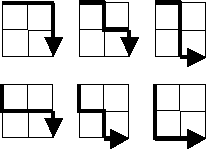## Project Euler Problem 15 Solution

Lattice paths

by {BetaProjects} | Project Euler & HackerRank

### Project Euler Problem 15 Statement

Starting at the top left corner of a 2×2 grid, there are 6 routes (without backtracking) to the bottom right corner.How many routes are there through a 20×20 grid?

### SolutionHere’s a similar question with more context.

"Starting at the top left corner of a grid, how many ways through town are possible using only south or east one–way streets and ending at the bottom right corner of the n×n grid?"

The valid paths for a 2×2 grid shown in the example are the discreet (unique) permutations of {R, R, D, D}. We can list these as: {R, R, D, D}, {R, D, R, D}, {R, D, D, R}, {D, R, R, D}, {D, R, D, R} and {D, D, R, R}. You must have 2 Rs (rights) and 2 Ds (downs), the order not being important, and as long as you start from the top–left corner you will always end at the bottom–right corner.

#### A 4×4 grid example

To find the number of paths in a 4×4 grid, you’ll have to generate all the discreet permutations of {R, R, R, R, D, D, D, D}, where any combination of 4 Rs and 4 Ds will always be a valid path. If the Rs are first placed randomly in any 4 of the 8 open positions, then they are considered independent. The Ds are considered dependent because they have no choice but to be placed into the remaining slots.

For example, placing the 4 Rs randomly in any of the available 8 positions as {R, _, R, R, _, R, _, _} will dictate where the Ds get placed as {R, D, R, R, D, R, D, D}. You will have 8C4 = 70 valid combinations. This could be interpreted as: how many distinct ways can you shuffle the characters in the string “RRRRDDDD”.

#### Count the number of routes using the binomial coefficient

The number of contiguous routes for a square grid (n×n) is the central binomial coefficient or the center number in the 2nth row of Pascal’s triangle.  The rows start their numbering at 0.The formula in general for any rectangular grid (n×m) using the notation for the binomial coefficient is:#### HackerRank version

HackerRank Project Euler 15 includes rectangular grids in addition to square grids, increases the size to 500×500 and runs 1,000 test cases.

### Python Source Code

``````from math import factorial as f
n, m = map(int, input("Enter dimensions (separate by space)?").split())
print ("Routes through a", n, "x", m, "grid", f(n+m) // f(n) // f(m))	``````Run Project Euler Problem 15 using Python on repl.it.

### Last Word

Another example assumes an 8 x 8 grid.

“Place a single rook on the top left corner of an empty chess board. Count the number of tours to the bottom right corner, moving only right and down.”

• Reference: The On–Line Encyclopedia of Integer Sequences (OEIS) A000984: Central binomial coefficients: C(2*n,n) = (2*n)!/(n!)^2.
• Changing the condition “contiguous paths which do not pass below the diagonal on an n×n grid” to our problem statement, we would use a Catalan number. It is calculated as: